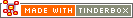sum(group, value)Operator Type: Boolean Operator Scope of Action: Group

The operator sum() adds lists. (See sum_if() for a related new operator)

sum(where,attribute)

visits each note described by where and adds the value of the designated attribute to the total.

where may be any of {child,descendent,sibling,ancestor,all}.

In addition, where may be argument that designates a particular (single) note other than this.

what can be any expression, but is typically an attribute.

For example,

`sum(child,\$WordCount)`

constructs the current word count of the children of the note.

 Up: Non-query Boolean operators Previous: every(group,value) Next: sum_if(group,condition,value)

[Last updated: 14 Dec 2009, using v5.0]